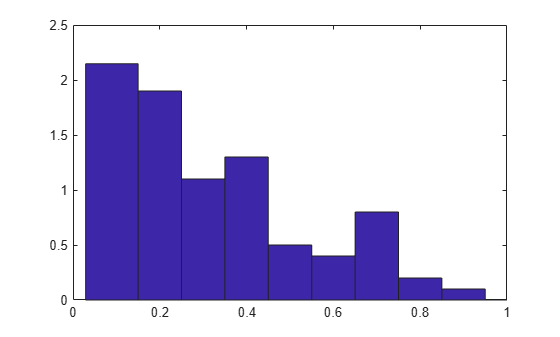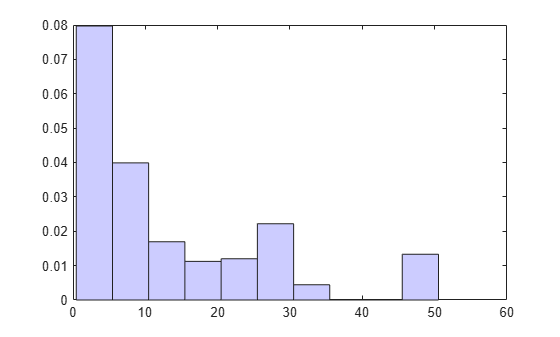ecdfhist

Histogram based on empirical cumulative distribution function

Description

example

[n,c] = ecdfhist(f,x) returns the heights, n, of histogram bars for 10 equally spaced bins and the position of the bin centers, c.

ecdfhist computes the bar heights from the increases in the empirical cumulative distribution function, f, at evaluation points, x. It normalizes the bar heights so that the area of the histogram is equal to 1. In contrast, histogram produces bars with heights representing bin counts.

example

[n,c] = ecdfhist(f,x,m) returns the histogram bars using m bins.

example

n = ecdfhist(f,x,centers) returns the heights of the histogram bars with bin centers specified by centers.

example

ecdfhist(___) plots the histogram bars.

Examples

collapse all

Compute the histogram bar heights based on the empirical cumulative distribution function.

Generate failure times from a Birnbaum-Saunders distribution.

rng('default') % for reproducibility
failuretime = random('birnbaumsaunders',0.3,1,100,1);

Assuming that the end of the study is at time 0.9, mark the generated failure times that are larger than 0.9 as censored data and store that information in a vector.

T = 0.9;
cens = (failuretime>T);

Compute the empirical cumulative distribution function for the data.

[f,x] = ecdf(failuretime,'censoring',cens);

Now, find the bar heights of the histogram using the cumulative distribution function estimate.

[n,c] = ecdfhist(f,x);
[n' c']
ans = 10×2

2.3529    0.0715
1.7647    0.1565
1.4117    0.2415
1.5294    0.3265
1.0588    0.4115
0.4706    0.4965
0.4706    0.5815
0.9412    0.6665
0.2353    0.7515
0.2353    0.8365

Compute the bar heights for six bins using the empirical cumulative distribution function and also return the bin centers.

Generate failure times from a Birnbaum-Saunders distribution.

rng('default') % for reproducibility
failuretime = random('birnbaumsaunders',0.3,1,100,1);

Assuming that the end of the study is at time 0.9, mark the generated failure times that are larger than 0.9 as censored data and store that information in a vector.

T = 0.9;
cens = (failuretime>T);

First, compute the empirical cumulative distribution function for the data.

[f,x] = ecdf(failuretime,'censoring',cens);

Now, estimate the histogram with six bins using the cumulative distribution function estimate.

[n,c] = ecdfhist(f,x,6);
[n' c']
ans = 6×2

1.9764    0.0998
1.7647    0.2415
1.1294    0.3831
0.4235    0.5248
0.7764    0.6665
0.2118    0.8081

Draw the histogram of the empirical cumulative distribution histogram for specified bin centers.

Generate failure times from a Birnbaum-Saunders distribution.

rng default;  % For reproducibility
failuretime = random('birnbaumsaunders',0.3,1,100,1);

Assuming that the end of the study is at time 0.9, mark the generated failure times that are larger than 0.9 as censored data and store that information in a vector.

T = 0.9;
cens = (failuretime>T);

Define bin centers.

centers = 0.1:0.1:1;

Compute the empirical cumulative distribution function for the data and draw the histogram for specified bin centers.

[f,x] = ecdf(failuretime,'censoring',cens);
ecdfhist(f,x,centers)
axis([0 1 0 2.5])Generate right-censored survival data and compare the histogram from cumulative distribution function with the known probability distribution function.

Generate failure times from an exponential distribution with mean failure time of 15.

rng default; % For reproducibility
y = exprnd(15,75,1);

Generate drop-out times from an exponential distribution with mean failure time of 30.

d = exprnd(30,75,1);

Record the minimum of these times as the observed failure times.

t = min(y,d);

Generate censoring by finding the generated failure times that are greater than the drop-out times.

censored = (y>d);

Calculate the empirical cdf and plot a histogram using the empirical cumulative distribution function.

[f,x] = ecdf(t,'censoring',censored);
ecdfhist(f,x)
h = findobj(gca,'Type','patch');
h.FaceColor = [.8 .8 1];
hold onSuperimpose a plot of the known population pdf.

xx = 0:.1:max(t);
yy = exp(-xx/15)/15;
plot(xx,yy,'r-','LineWidth',2)
hold offInput Arguments

collapse all

Empirical cdf values at given evaluation points, x, specified as a vector.

For instance, you can use ecdf to obtain the empirical cdf values and enter them in ecdfhist as follows.

Example: [f,x] = ecdf(failure); ecdfhist(f,x);

Data Types: single | double

Evaluation points at which empirical cdf values, f, are calculated, specified as a vector.

For instance, you can use ecdf to obtain the empirical cdf values and enter them in ecdfhist as follows.

Example: [f,x] = ecdf(failure); ecdfhist(f,x);

Data Types: single | double

Number of bins, specified as a scalar.

For instance, you can draw a histogram with 8 bins as follows.

Example: ecdfhist(f,x,8)

Data Types: single | double

Center points of bins, specified as a vector.

Example: centers = 2:2:10; ecdfhist(f,x,centers);

Data Types: single | double

Output Arguments

collapse all

Heights of histogram bars ecdfhist calculates based on the empirical cdf values, returned as a row vector.

Position of bin centers, returned as a row vector.